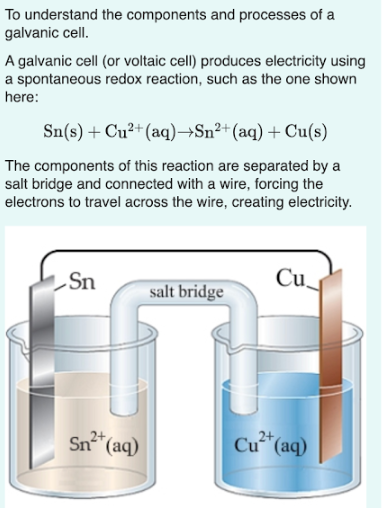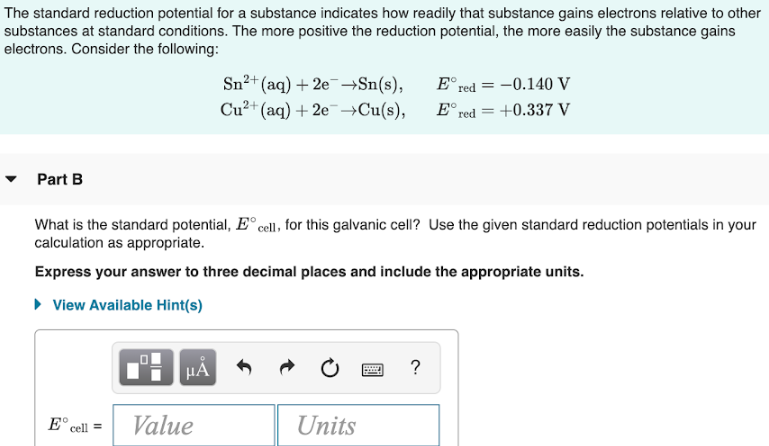# To understand the components and processes of a galvanic cell. A galvanic cell (or voltaic cell) produces electricity using a spontaneous redox reaction, such as the one shown here: Sn(s) + Cu^2+(aq) → Sn^2+(aq) + Cu(s) The components of this reaction are separated by a salt bridge and connected with a wire, forcing the electrons to travel across the wire, creating electricity. The salt bridge is a U-shaped glass tube that is filled with a gel-like substance containing a salt. The salt bridge completes the circuit and allows ion to flow, maintaining electrical neutrality in the solutions. The standard reduction potential for a substance indicates how readily that substance gains electrons relative to other substances at standard conditions. The more positive the reduction potential, the more easily the substance gains electrons. Consider the following: Sn^2+(aq) + 2e^- → Sn(s), E° red = -0.140 V Cu^2+(aq) + 2e^- → Cu(s), E° red = +0.337 V What is the standard potential, E° cell, for this galvanic cell? Use the given standard reduction potentials in your calculations as appropriate. Express your answer to three decimal places and include the appropriate units.